Courses

# Deflection And Theories Of Failure - 1

## 10 Questions MCQ Test Topicwise Question Bank for GATE Mechanical Engineering | Deflection And Theories Of Failure - 1

Description
This mock test of Deflection And Theories Of Failure - 1 for Mechanical Engineering helps you for every Mechanical Engineering entrance exam. This contains 10 Multiple Choice Questions for Mechanical Engineering Deflection And Theories Of Failure - 1 (mcq) to study with solutions a complete question bank. The solved questions answers in this Deflection And Theories Of Failure - 1 quiz give you a good mix of easy questions and tough questions. Mechanical Engineering students definitely take this Deflection And Theories Of Failure - 1 exercise for a better result in the exam. You can find other Deflection And Theories Of Failure - 1 extra questions, long questions & short questions for Mechanical Engineering on EduRev as well by searching above.
QUESTION: 1

Solution:
QUESTION: 2

### A simply supported beam carries a concentrated load P at the mid span and experiences a maximum deflection Av Subsequently, the breadth and depth of beam is doubled. The corresponding deflection under the load will become

Solution: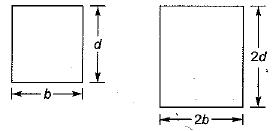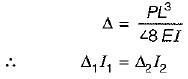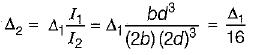QUESTION: 3

### A simply supported beam carries a total load W which is uniformly distributed on the entire span. The center of beam is propped so that it is brought to the level of end supports. The prop reaction would be

Solution:

After equating central deflection for both the cases, we have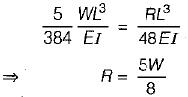QUESTION: 4

A point load placed at the mid span of a simply supported beam has been replaced by a uniformly distributed load of same total value. The central deflection in the later case will be

Solution: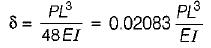For udl of same total value,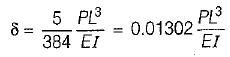QUESTION: 5

A cantilevel beam of length L is subjected to a concentrated load Pat a distance of L/3 from the free end. What is the deflection at free end of the beam?

Solution:
QUESTION: 6

Which amongst the following methods is/are most commonly used to determine beam deflection?
1. Double-integration method
2. Method of singularity functions
3. Elastic energy methods
4. Moment-area methods

Solution:
QUESTION: 7

The differential equation of the bent beam is given by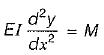The significance of this equation is, except

Solution:
QUESTION: 8

A support is said to be non-yielding if

Solution:
QUESTION: 9

A simply supported beam has been subjected to unsymmetrical loading. The deflection would be maximum at a section where

Solution:

Slope is always zero at the point of maximum deflection.

QUESTION: 10

Which of the following statements pertaining to slope of loaded beam is WRONG? The maximum slope

Solution:

The maximum slope for a simply supported beam carrying a total load Pwhich is distributed over the entire span is  PL2/24 EI